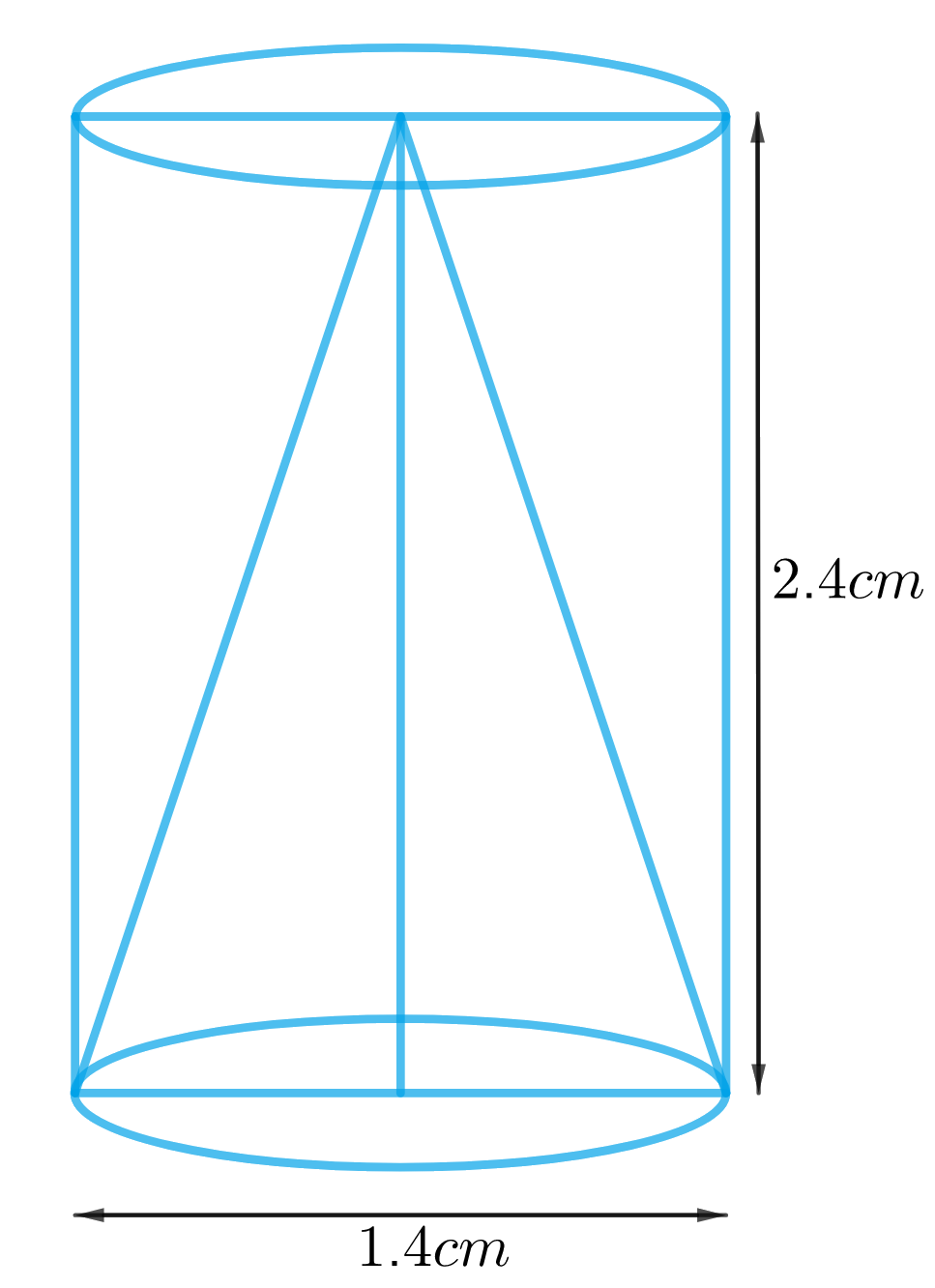# Ex.13.1 Q8 Surface Areas and Volumes Solution - NCERT Maths Class 10

Go back to  'Ex.13.1'

## Question

From a solid cylinder whose height is $$2.4 \,\rm{cm}$$ and diameter $$1.4\,\rm{ cm}$$, a conical cavity of the same height and same diameter is hollowed out. Find the total surface area of the remaining solid to the nearest $$\rm{cm}^2$$.

Video Solution
Surface Areas And Volumes
Ex 13.1 | Question 8

## Text Solution

What is known?

A solid cylinder of height $$2.4 \,\rm{cm}$$ and diameter $$1.4\,\rm{ cm}$$ from which a conical cavity of the same height and same diameter is hollowed out.

What is unknown?

Total surface area of the remaining solid.

Reasoning:

We can create the figure of the solid as per given informationSince the conical cavity of the same diameter has been hollowed out, its clear that one of the bases of the cylinder is not included in total surface area of solid.

TSA of the remaining solid $$=$$ CSA of the cylindrical part $$+$$ CSA of conical part $$+$$ Area of a cylinderical base

We will find the area of the remaining solid by using formulae;

CSA of the cylinder  $$= 2\pi rh$$
Area of the base of the cylinder  $$= \pi {r^2}$$

where $$r$$ and $$h$$ are radius and height of the cylinder respectively.
CSA of the cone $$= \pi rl$$

Slant height of the cone, $$l = \sqrt {{r^2} + {h^2}}$$
where $$r, h$$ and $$l$$ are radius, height and slant height of the cone respectively.

Steps:

Height of the cylinder $$=$$ Height of the cone $$= h = 2.4 \rm cm$$

Diameter of the cylinder $$=$$ diameter of the cone $$= d = 1.4 \rm cm$$

Radius of the cylinder $$=$$ radius of the cone \begin{align} = r = \frac{{1.4 \rm cm}}{2} = 0.7 \rm cm\end{align}

Slant height of the cone,$$l = \sqrt {{r^2} + {h^2}}$$

\begin{align}l &= \sqrt {{{\left( {0.7\rm cm} \right)}^2} + {{\left( {2.4cm} \right)}^2}} \\ &= \sqrt {0.49 \rm c{m^2} + 5.76c{m^2}} \\&= \sqrt {6.25 \rm c{m^2}} \\&= 2.5 \rm cm\end{align}

TSA of the remaining solid $$=$$ CSA of the cylindrical part $$+$$ CSA of conical part $$+$$Area of the cylindrical base

\begin{align}&= 2\pi rh + \pi rl + \pi {r^2}\\& = \pi r\left( {2h + l + r} \right)\\&= \!\!\frac{{22}}{7}\! \!\times\! 0.7 \rm cm \!\times\!\! ( 2 \!\times\! 2.4 \rm cm \!+ \! 2.5 \rm cm \!+\! 0.7cm ) \\&= 2.2 \rm cm \times 8cm\\&= 17.6 \rm c{m^2}\end{align}

Hence, the total surface area of the remaining solid to the nearest $$\rm cm^2$$ is $$18 \, \rm cm^2.$$

Learn from the best math teachers and top your exams

• Live one on one classroom and doubt clearing
• Practice worksheets in and after class for conceptual clarity
• Personalized curriculum to keep up with school